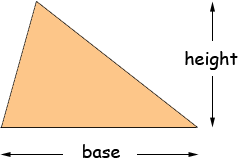ok um my question is how do you find the area of a triangle? There are two standard ways to find the area of a triangle, which you use depends on what you know about the triangle. If you know the length of one side (call it the base) and the height of the triangle you can find the area usingarea = 1/2 baseheight If you know the lengths of the three sides of the triangle you can find its area using Heron's Formula. I hope this helps, Penny